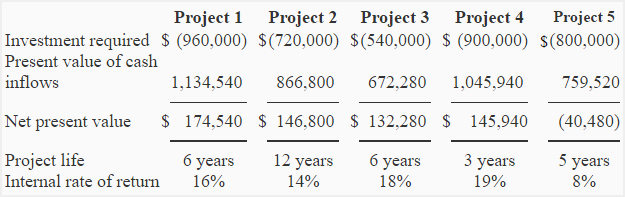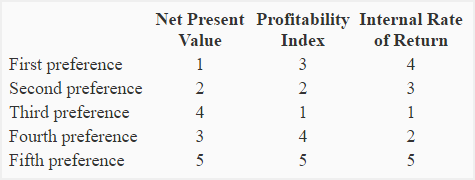# Problem-4 (Preference ranking of investment projects)

The Martin Company is considering the four different investment opportunities. The selected information about each proposal is given below:The present value of cash inflows given above have been computed using a 10% discount rate. The company is unable to accept all available projects because the funds available for investment are limited.

Required:

1. Compute the profitability index (present value index) for all the projects.
2. Rank the four investment projects according to preference using:
(a). net present value (NPV).
(b). profitability index (PI).
(c). internal rate of return (IRR).
3. Which one is the best approach for Martin Company to rank five competing projects?

## Solution:

### (1). Computation of profitability index:

Formula of profitability/present value index is:

Profitability index = Present value of cash inflows/Investment required

Project 1: \$1,134,540/\$960,000 = 1.18

Project 2: \$866,800/\$720,000 = 1.20

Project 3: \$672,280/\$540,000 = 1.24

Project 4: \$1,045,490/\$900,000 = 1.16

Project 5: \$759,520/\$800,000 = 0.95

### (2) Preference ranking of projects:### 3. The best ranking approach:

The best method of ranking projects depends on the availability of good reinvestment opportunities. Under internal rate of return (IRR) method, we assume that the funds released from a project are reinvested in another project yielding the internal rate of return equal to the previous project. According to IRR, the project 4 is ranked at number one with 19% IRR. It means any funds released from project 4 must be reinvested in another project yielding an internal rate of return of at least 19% but It might be difficult to find a project with such a high IRR.

The profitability index (PI) shows the present value of cash inflow generated by each dollar invested in a project. It assumes that the funds released from a project are reinvested in another project with a return equal to the discount rate. In our problem, the discount rate is only 10%. Generally, the profitability index is considered the most dependable method of ranking competing projects.

The net present value (NPV) method considers the net present value figure but does not take into account the amount of investment required for the project. Therefore, this method is not appropriate for comparing or ranking competing projects that require different amounts of investment.  For example, project 3 is ranked at number four because of its low net present value but it is the best option if we see at the present value of net cash inflow generated by each dollar invested in the project (as shown by the profitability index).

Conclusion: From above discussion, we can conclude that the profitability index is the most appropriate and dependable method of ranking projects for Martin Company.

A D V E R T I S E M E N T
One Comment on Problem-4 (Preference ranking of investment projects)
1.MD. Mamun

If the company has investable funds only at the amount of \$ 2,500,000 how it should invest the funds among these projects.